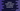# Python program to find the middle element of a random number list## Python program to find the middle element of a random number list :

In this python programming tutorial, we will learn how to find out the mid number in a number list. For example, if the list is [1,6,5,4,3], the mid element is 4. Because two numbers 1,3 are less than 4 and two elements 5,6 are greater than 4. Our list will contain an odd number of elements.

### Algorithm :

You can solve this problem by iterating through each element of the list one by one and testing each element if it is the middle element or not. We can check it by comparing the count of all smaller and bigger elements in the list. If for a number, the list contains an equal amount of smaller and bigger numbers, it will be the middle number in that list. The main problem of this solution is that we need to iterate through the list multiple times.

Another way and the most preferred way to solve this problem is by sorting the list. If we sort the list, it will move the middle element to the centre. Using the length of the list, we can find out the mid element easily.

## Sorting a list in python :

Python comes with one inbuilt method ‘sort()’ to sort elements of a list in ascending or descending order. It doesn’t take any parameter but optionally you can pass one parameter ‘reverse’ to sort the list in reversed order.

If ‘reverse’ is ’True’, the list will be sorted in reversed order. Else, it will sort the list in increasing order, which is the default behavior.

sort() method doesn’t return any value. It will modify the original list.

In this example, we will sort the list in default order. Actually, we can sort it in any order we want. The mid element will be placed always in the middle if the list is sorted.

### Python program :

``````#1
my_list = [4,3,2,9,10,44,1]
#2
my_list.sort()
#3
print("sorted list is ",my_list)
#4
print("mid value is ",my_list[int(len(my_list)/2)])``````